# Spectrum

A spectrum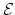is (roughly) a sequence of based spaces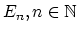, provided with maps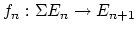(where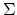denotes suspension). There are many different definitions of the category of spectra, but they all yield the same homotopy category, known as the stable homotopy category. The homotopy category of spectra forms a triangulated category (with shifts given by suspension and looping); if we associate to a space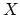the suspesion spectrum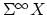with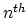-space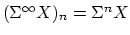, the homotopy classes of maps between the suspension spectra ofand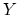are the stable homotopy classes of maps betweenand. There is a correspondence between generalized (co)homology theories and spectra as follows. Given a generalized cohomology theory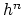, the Brown representability theorem gives a (universal) space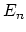such that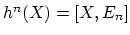; the suspension axiom provides the required structure maps forto form a spectrum. Conversely, for any spectrum, the functor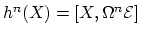is a generalized cohomology theory, and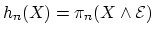is a generalized homology theory.

Jeffrey Herschel Giansiracusa 2005-06-27